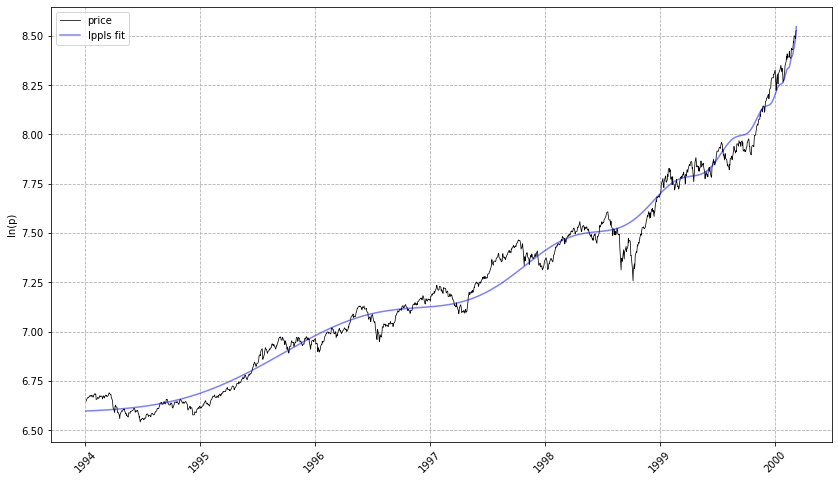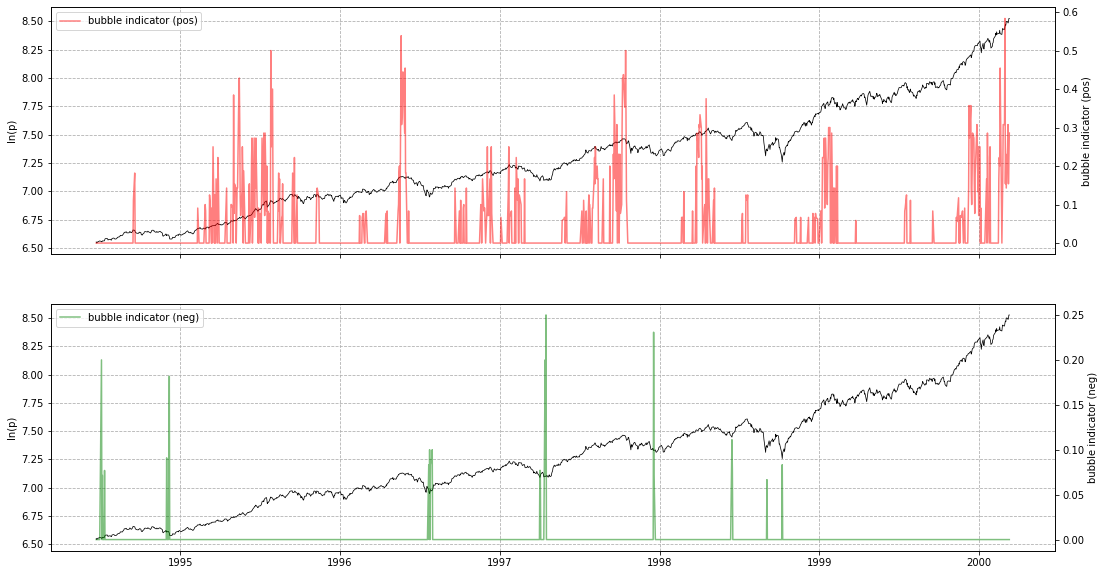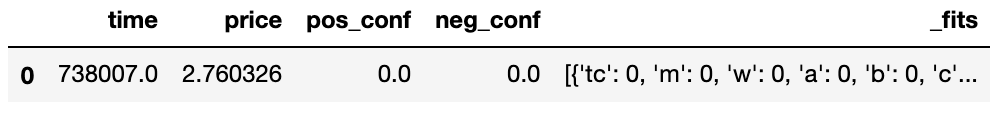lppls
pypi i lppls

# lppls

## Library for fitting the LPPLS model to data.

### by Boulder-Investment-Technologies

0.6.5 (see all)
pypi i lppls# Log Periodic Power Law Singularity (LPPLS) Model

`lppls` is a Python module for fitting the LPPLS model to data.

## Overview

The LPPLS model provides a flexible framework to detect bubbles and predict regime changes of a financial asset. A bubble is defined as a faster-than-exponential increase in asset price, that reflects positive feedback loop of higher return anticipations competing with negative feedback spirals of crash expectations. It models a bubble price as a power law with a finite-time singularity decorated by oscillations with a frequency increasing with time.

Here is the model:where:

•expected log price at the date of the termination of the bubble
•critical time (date of termination of the bubble and transition in a new regime)
•expected log price at the peak when the end of the bubble is reached at•amplitude of the power law acceleration
•amplitude of the log-periodic oscillations
•degree of the super exponential growth
•scaling ratio of the temporal hierarchy of oscillations
•time scale of the oscillations

The model has three components representing a bubble. The first,, handles the hyperbolic power law. For< 1 when the price growth becomes unsustainable, and atthe growth rate becomes infinite. The second term,, controls the amplitude of the oscillations. It drops to zero at the critical time. The third term,, models the frequency of the osciallations. They become infinite at.

## Installation

Dependencies

`lppls` requires:

• Python (>= 3.7)
• Matplotlib (>= 3.1.1)
• Numba (>= 0.51.2)
• NumPy (>= 1.17.0)
• Pandas (>= 0.25.0)
• SciPy (>= 1.3.0)
• Pytest (>= 6.2.1)

User installation

``````pip install -U lppls
``````

## Example Use

``````from lppls import lppls, data_loader
import numpy as np
import pandas as pd
from datetime import datetime as dt
%matplotlib inline

# read example dataset into df

# convert time to ordinal
time = [pd.Timestamp.toordinal(dt.strptime(t1, '%Y-%m-%d')) for t1 in data['Date']]

# create list of observation data

# create observations array (expected format for LPPLS observations)
observations = np.array([time, price])

# set the max number for searches to perform before giving-up
# the literature suggests 25
MAX_SEARCHES = 25

# instantiate a new LPPLS model with the Nasdaq Dot-com bubble dataset
lppls_model = lppls.LPPLS(observations=observations)

# fit the model to the data and get back the params
tc, m, w, a, b, c, c1, c2, O, D = lppls_model.fit(MAX_SEARCHES)

# visualize the fit
lppls_model.plot_fit()

# should give a plot like the following...
````````````# compute the confidence indicator
res = lppls_model.mp_compute_nested_fits(
workers=8,
window_size=120,
smallest_window_size=30,
outer_increment=1,
inner_increment=5,
max_searches=25,
# filter_conditions_config={} # not implemented in 0.6.x
)

lppls_model.plot_confidence_indicators(res)
# should give a plot like the following...
``````If you wish to store `res` as a pd.DataFrame, use `compute_indicators`.

Example
``````res_df = lppls_model.compute_indicators(res)
res_df
# gives the following...
``````## Other Search Algorithms

Shu and Zhu (2019) proposed CMA-ES for identifying the best estimation of the three non-linear parameters (,,).

The CMA-ES rates among the most successful evolutionary algorithms for real-valued single-objective optimization and is typically applied to difficult nonlinear non-convex black-box optimization problems in continuous domain and search space dimensions between three and a hundred. Parallel computing is adopted to expedite the fitting process drastically.

This approach has been implemented in a subclass and can be used as follows... Thanks to @paulogonc for the code.

``````from lppls import lppls_cmaes
lppls_model = lppls_cmaes.LPPLSCMAES(observations=observations)
tc, m, w, a, b, c, c1, c2, O, D = lppls_model.fit(max_iteration=2500, pop_size=4)
``````

Performance Note: this works well for single fits but can take a long time for computing the confidence indicators. More work needs to be done to speed it up.

• Filimonov, V. and Sornette, D. A Stable and Robust Calibration Scheme of the Log-Periodic Power Law Model. Physica A: Statistical Mechanics and its Applications. 2013
• Shu, M. and Zhu, W. Real-time Prediction of Bitcoin Bubble Crashes. 2019.
• Sornette, D. Why Stock Markets Crash: Critical Events in Complex Financial Systems. Princeton University Press. 2002.
• Sornette, D. and Demos, G. and Zhang, Q. and Cauwels, P. and Filimonov, V. and Zhang, Q., Real-Time Prediction and Post-Mortem Analysis of the Shanghai 2015 Stock Market Bubble and Crash (August 6, 2015). Swiss Finance Institute Research Paper No. 15-31.

181

8mos ago

1

10

4

### OPEN PRs

2
VersionTagPublished
0.6.5
2mos ago
0.6.4
8mos ago
0.6.3
8mos ago
0.6.2
8mos ago